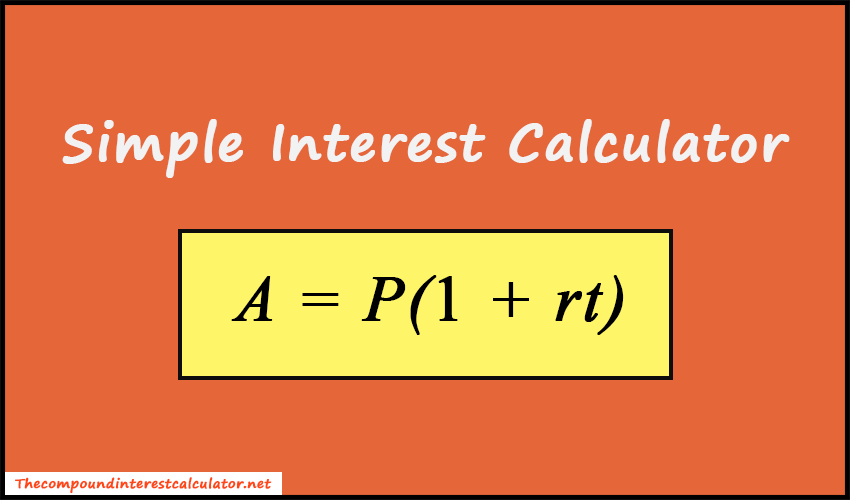# Simple Interest Calculator

Simple Interest Calculator helps to calculate the interest amount using principal amount, annual interest rate, and time period.

\$
%
Compound Interest Calculator Average Calculator

Simple Interest Calculator is a free financial tool where you can find the interest amount and total amount instantly. Also, you don't need to do any type of manual calculations. Just enter the principal amount, annual interest rate, and the time period(in days, weeks, months, quarters, and years) and press the Calculate button. As a result, the tool will display the interest amount and total amount within a second.### Simple Interest FormulaWhere,

A = Final amount (principal + interest)
P = Principal amount
r = Annual interest rate (decimal)
t = Time (in years)